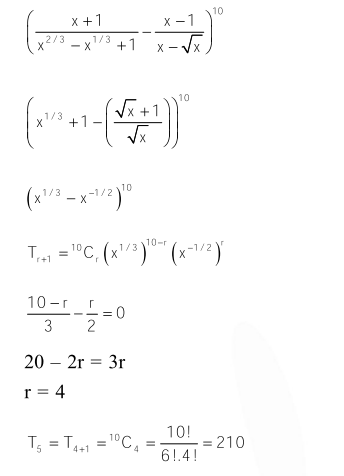# The term independent of x in expansion of`
Question:

The term independent of $x$ in expansion of $\left(\frac{x+1}{x^{2 / 3}-x^{1 / 3}+1}-\frac{x-1}{x-x^{1 / 2}}\right)^{10}$ is :

1.  4

2. 120

3. 210

4. 310

Correct Option: , 3

Solution: